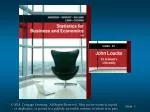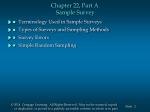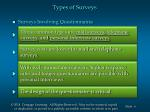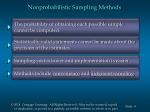Download PresentationJohn Loucks St . Edward’s University

# John Loucks St . Edward’s University - PowerPoint PPT Presentation

SLIDES . BY. . . . . . . . . . . . John Loucks St . Edward’s University. Chapter 22, Part A Sample Survey. Terminology Used in Sample Surveys . Types of Surveys and Sampling Methods. Survey Errors. Simple Random Sampling. Terminology Used in Sample Surveys.I am the owner, or an agent authorized to act on behalf of the owner, of the copyrighted work described.
Download Presentation## John Loucks St . Edward’s University

An Image/Link below is provided (as is) to download presentation

Download Policy: Content on the Website is provided to you AS IS for your information and personal use and may not be sold / licensed / shared on other websites without getting consent from its author.While downloading, if for some reason you are not able to download a presentation, the publisher may have deleted the file from their server.

- - - - - - - - - - - - - - - - - - - - - - - - - - E N D - - - - - - - - - - - - - - - - - - - - - - - - - -
Presentation TranscriptSLIDES.BY

.

.

.

.

.

.

.

.

.

.

.

John Loucks

St. Edward’s

UniversityChapter 22, Part A Sample Survey
• Terminology Used in Sample Surveys
• Types of Surveys and Sampling Methods
• Survey Errors
• Simple Random SamplingTerminology Used in Sample Surveys

An element is the entity on which data are collected.

A population is the collection of all elements of interest.

A sample is a subset of the population.Terminology Used in Sample Surveys

The target populationis the population we want to

The sampled populationis the population from which

the sample is actually selected.

These two populations are not always the same.

If inferences from a sample are to be valid, the sampled

population must be representative of the target

population.Terminology Used in Sample Surveys

The population is divided into sampling unitswhich

are groups of elements or the elements themselves.

A list of the sampling units for a particular study is

called a frame.

The choice of a particular frame is often determined by

the availability and reliability of a list.

The development of a frame can be the most difficult

and important steps in conducting a sample survey.Types of Surveys

• Surveys Involving Questionnaires

Three common types are mail surveys, telephone

surveys, and personal interview surveys.

Survey costs are lower for mail and telephone

surveys.

With well-trained interviewers, higher response

rates and longer questionnaires are possible with

personal interviews.

The design of the questionnaire is critical.Types of Surveys

• Surveys Not Involving Questionnaires

Often, someone simply counts or measures the

sampled items and records the results.

An example is sampling a company’s inventory of

parts to estimate the total inventory value.Sampling Methods

Sample surveys can also be classified in terms of the

sampling method used.

The two categories of sampling methods are:

Nonprobabilistic sampling

Probabilistic samplingNonprobabilistic Sampling Methods

The probability of obtaining each possible sample

cannot be computed.

Statistically valid statements cannot be made about the

precision of the estimates.

Sampling cost is lower and implementation is easier.

Methods include convenience and judgment sampling.Nonprobabilistic Sampling Methods

• Convenience Sampling

The units included in the sample are chosen

because of accessibility.

In some cases, convenience sampling is the only

practical approach.Nonprobabilistic Sampling Methods

• Judgment Sampling

A knowledgeable person selects sampling units

that he/she feels are most representative of the

population.

The quality of the result is dependent on the person

selecting the sample.

Generally, no statistical statement should be made

about the precision of the result.Probabilistic Sampling Methods

The probability of obtaining each possible sample

can be computed.

Confidence intervals can be developed which provide

bounds on the sampling error.

Methods include simple random, stratified simple

random, cluster, and systematic sampling.Survey Errors

Two types of errors can occur in conducting a survey :

Sampling error

Nonsampling errorSurvey Errors

• Sampling Error

It is defined as the magnitude of the difference

between the point estimate, developed from the

sample, and the population parameter.

It occurs because not every element in the

population is surveyed.

It cannot occur in a census.

It can not be avoided, but it can be controlled.Survey Errors

• Nonsampling Error

It can occur in both a census and a sample survey.

Examples include:

Measurement error

Errors due to nonresponse

Errors due to lack of respondent knowledge

Selection error

Processing errorSurvey Errors

• Nonsampling Error
• Measurement Error

Measuring instruments are not properly

calibrated.

People taking the measurements are not properly

trained.Survey Errors

• Nonsampling Error
• Errors Due to Nonresponse

They occur when no data can be obtained, or

only partial data are obtained, for some of the

units surveyed.

The problem is most serious when a bias is

created.Survey Errors

• Nonsampling Error
• Errors Due to Lack of Respondent Knowledge

These errors on common in technical surveys.

Some respondents might be more capable than

others of answering technical questions.Survey Errors

• Nonsampling Error
• Selection Error

An inappropriate item is included in the survey.

For example, in a survey of “small truck owners”

some interviewers include SUV owners while

other interviewers do not.Survey Errors

• Nonsampling Error
• Processing Error

Data is incorrectly recorded.

Data is incorrectly transferred from recording

forms to computer files.Simple Random Sampling

A simple random sampleof size n from a finite

population of size N is a sample selected such that

every possible sample of size n has the same

probability of being selected.

We begin by developing a frameor list of all

elements in the population.

Then a selection procedure, based on the use of

random numbers, is used to ensure that each

element in the sampled population has the same

probability of being selected.Simple Random Sampling

We will see in the upcoming slides how to:

• Estimate the following population parameters:
• Population mean
• Population total
• Population proportion
• Determine the appropriate sample sizeSimple Random Sampling

In a sample survey it is common practice to provide

an approximate 95% confidence interval estimate of

the population parameter.

Assuming the sampling distribution of the point

estimator can be approximated by a normal

probability distribution, we use a value of t = 2 for

a 95% confidence interval.Simple Random Sampling

• The interval estimate is:

Point Estimator +/-

2(Estimate of the Standard Error of the Point Estimator)

• The bound on the sampling error is:

2(Estimate of the Standard Error of the Point Estimator)Simple Random Sampling

• Population Mean
• Point Estimator
• Estimate of the Standard Error of the MeanSimple Random Sampling

• Population Mean
• Interval Estimate
• Approximate 95% Confidence Interval EstimateSimple Random Sampling
• Estimate of the Standard Error of the Total
• Population Total
• Point EstimatorSimple Random Sampling

• Population Total
• Interval Estimate
• Approximate 95% Confidence Interval EstimateSimple Random Sampling

• Population Proportion
• Point Estimator
• Estimate of the Standard Error of the ProportionSimple Random Sampling

• Population Proportion
• Interval Estimate
• Approximate 95% Confidence Interval EstimateDetermining the Sample Size

An important consideration is choice of sample size.

The best choice usually involves a tradeoff between

cost and precision (size of the confidence interval).

Larger samples provide greater precision, but are

more costly.

A budget might dictate how large the sample can be.

A specified level of precision might dictate how

small a sample can be.Determining the Sample Size

Smaller confidence intervals provide more precision.

The size of the approximate confidence interval

depends on the bound B on the sampling error.

Choosing a level of precision amounts to choosing a

value for B.

Given a desired level of precision, we can solve for

the value of n.Simple Random Sampling
• Necessary Sample Size

for Estimating the Population Mean

Hence,Simple Random Sampling
• Necessary Sample Size

for Estimating the Population TotalSimple Random Sampling
• Necessary Sample Size

for Estimating the Population ProportionSimple Random Sampling
• Example: Steddy Investments

Ben Steddy is a financial advisor for 200 clients.

A sample of 40 clients has been taken to obtain

various demographic data and information about the

clients’ investment objectives. Statistics of particular

interest are the clients’ net worth and the proportion

favoring fixed income investments.Simple Random Sampling
• Example: Steddy Investments

For the sample, the mean net worth was \$480,000

(with a standard deviation of \$120,000), and the

proportion favoring fixed-income investments was

.30.Simple Random Sampling
• Point Estimate of Total Net Worth (TNW)
• Approximate 95% Confidence Interval for TNW
• Estimate of Standard Error of TNW

= \$3,394,113

= \$89,211,774 to \$102,788,226Simple Random Sampling

• Point Estimate of Population Proportion

Favoring Fixed-Income Investments

• Estimate of Standard Error of Proportion
• Approximate 95% Confidence IntervalSimple Random Sampling

One year later Steddy wants to again survey his

clients. He now has 250 clients and wants to set a

bound of \$30,000 on the error of the estimate of

their mean net worth.

What is the necessary sample size?

• Example: Steddy InvestmentsExample: Steddy Investments

• Necessary Sample Size

Steddy will need a sample size of 51.